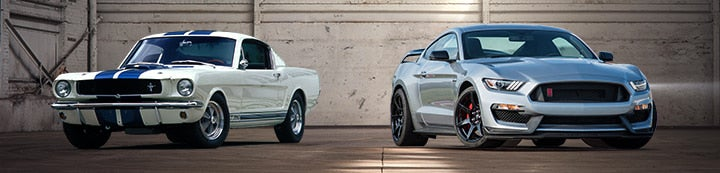1 - 7 of 7 Posts

#### stvitus2002

·
##### Registered
Joined
·
2 Posts
Discussion Starter · ·
I have a formula for calculating cubic
inches-
Bore x 3.14(pie)=result x stroke=result x
No. of cyl=Cubic inches.

Example: 302- 4.00 x 3.14=12.56 X 3.00=37.68 x 8=301.44 Cu in
This formula works for the 302,289(288.3776),351(351.6
.
But the 429 comes in at 393.3696??
The 460 is a 421.8584-quite a difference!
This is assuming a bore of 4.362 for both the 429 & 460. Stroke of 3.59 for the 429
& stroke of 3.85 for the 460.
Am i missing something or is this a case

#### TurboCoupe50

·
##### Registered
Joined
·
809 Posts
I believe NHRA uses this formula.

Bore x Bore x Stroke x .7854 x number of cylinders

#### Tim R

·
##### Registered
Joined
·
165 Posts
here is one that I use and it works every time and it is right on the money

bore x bore x stroke x number of cylinders x .7854

example 460BBF

4.360 x 4.360 x 3.85 x 8 x .7854 = 459.8483

easy to remember and very close every time try it out.

#### stvitus2002

·
##### Registered
Joined
·
2 Posts
Discussion Starter · ·
Got It! Thanks.

#### BOB PHILLIPS

·
##### Registered
Joined
·
114 Posts
Try this, it is much simpler for (V 8 only)
bore x bore x stroke x 6.2832

#### MightyMach

·
##### Registered
Joined
·
2,525 Posts
Try PiR^2 xHeight x Cylinders Reads Pi x Radius squared x Stroke x Cylinders. Pi is approx. 3.14

Jeff Given

#### tonyk

·
##### Registered
Joined
·
280 Posts
Your flaw comes from doing PI x bore diam.
Bore diam x PI give circumference of bore.
What you want is area of bore.
You need PI x radius squared.
ie 3.1415 x (bore diam/2)^2
ie for a 302 its OK cos its 4 inch and half 4 inch squared is 4 inch, but when its 4.2 inch its 2.1 x 2.1 = 4.41.
Thats where you 429 / 460 started to come out wrong.

On 2002-02-26 21:57, stvitus2002 wrote:
I have a formula for calculating cubic
inches-
Bore x 3.14(pie)=result x stroke=result x
No. of cyl=Cubic inches.

Example: 302- 4.00 x 3.14=12.56 X 3.00=37.68 x 8=301.44 Cu in
This formula works for the 302,289(288.3776),351(351.6
.
But the 429 comes in at 393.3696??
The 460 is a 421.8584-quite a difference!
This is assuming a bore of 4.362 for both the 429 & 460. Stroke of 3.59 for the 429
& stroke of 3.85 for the 460.
Am i missing something or is this a case